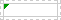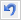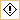# How to Detect Errors in Formulas

• Applies to: Excel for Microsoft 365, Excel for Microsoft 365 for Mac, Excel 2019, Excel 2016, Excel 2019 for Mac, Excel 2013, Excel 2010, Excel 2007, Excel 2016 for Mac, Excel for Mac 2011, Excel Starter 2010.

Formulas can often cause error values as well as gathering unexpected results. The following are some tools that you can use for finding and correcting formula errors then analysing their causes, and identifying solutions.

Note: This topic includes techniques that can guide you with finding and correcting formula errors. It is not an exhaustive list of methods for correcting every possible formula error. For help on specific errors, you can search for questions like yours in the Excel Community Forum, or post one of your own.

## Learn how to enter a simple formula

Formulas are equations that perform calculations on values in your worksheet. A formula starts with an equal sign (=). For example, the following formula adds 3 to 1.

=3+1

A formula can also contain any or all of the following: functions, references, operators, and constants.

## Parts of a formula

1. Functions: built-in with Excel, functions are formulas that perform specific calculations due to its articulate design. For example, the PI() function returns the value of pi: 3.142…
2. References: refer to individual cells or ranges of cells. A2 returns the value in cell A2.
3. Constants: numbers or text values entered directly into a formula, such as 2.
4. Operators: The ^ (caret) operator raises a number to a power, and the * (asterisk) operator multiplies. Use + and – add and subtract values, and / to divide.

Note: Some functions require arguments. Arguments are the values that certain functions use to perform their calculations. Arguments reside between the function’s parentheses () any time they must be present. The PI function does not require any arguments, which is why it’s blank. Some functions require one or more arguments, and can leave room for additional arguments. You need to use a comma to separate arguments, or a semi-colon (;) depending on your location settings.

The SUM function for example, requires only one argument, but can accommodate 255 total arguments.

=SUM(A1:A10) is an example of a single argument.

=SUM(A1:A10, C1:C10) is an example of multiple arguments.

## Finding and correcting common formula errors when entering formulas

The following table summarises some of the most common errors that a user can make when entering a formula, and explains how to correct them.

## Finding and correcting formula errors – common problems in formulas

You can enforce specific rules for finding and correcting errors in formulas. These rules however, do not fully confirm that your worksheet is infallible, but nevertheless, they are huge timesavers in helping you to find common mistakes. You can enable and disable any of these rules individually.

There are two ways to highlight and correct errors: one error at a time (like a spell checker), or immediately during any of their occurrences on the worksheet as you enter data.

You can resolve an error by selecting Excel’s built-in options, or you can ignore the error by selecting Ignore Error. If you ignore an error in a particular cell, the error in that cell will refrain from displaying in pending error checks. However, you can reset all past ignored errors so that they display again.

## Turn error checking rules on or off

1. For Excel on Windows, press File > Options > Formulas, or
for Excel on Mac, select the Excel menu > Preferences > Error Checking. In Excel 2007, click the Microsoft Office button> Excel Options > Formulas.
2. Below Error Checking, check Enable background error checking. Any found error will include a triangle in the top-left corner of the cell.3. To edit the colour of the triangle that marks where an error occurs, in the Indicate errors using this colour box, pick your desired colour.
4. Below Excel checking rules, choose or clear the checkboxes of any of the following rules:

### Cells containing formulas that result in an error

• A formula omits using the expected syntax, arguments, or data types. Error values comprise #DIV/0!, #N/A, #NAME?, #NULL!, #NUM!, #REF!, and #VALUE!. Each of these error values have different causes and solutions.

### Important Note

Note: If you enter an error value straight into a cell, it takes the place of that error value but is not actually an error. However, if a formula in another cell refers to that cell, the formula returns the error value from that cell.

### Inconsistent calculated column formula in tables

• A calculated column can have individual formulas that contrast from the master column formula, which produces an exception. Calculated column exceptions arise when you do any of the following:

• Write data other than a formula in a calculated column cell.
• Input a formula in a calculated column cell, and then use Ctrl +Z or click Undoon the Quick Access Toolbar.
• Enter a new formula in a calculated column that already occupies one or more exceptions.
• Copy data into the calculated column that differs from the calculated column formula. If the copied data has a formula, this formula replaces the data in the calculated column.
• Reposition or erase a cell on another worksheet area that is directly referenced by one of the rows in a calculated column.

### Cells containing years represented as 2 digits

• The cell includes a text date that can be misinterpreted as the incorrect century once it is used in formulas. For example, the date in the formula =YEAR(“1/1/31”) could be 1931 or 2031. Use this rule to scan for vague text dates.

### Numbers formatted as text or preceded by an apostrophe

• The cell has numbers stored as text. This usually happens when data is imported from other sources. Numbers that are categorised as text can result in unexpected sorting results, so it is recommended to convert them to numbers. ‘=SUM(A1:A10) is classed as text.

### Formulas inconsistent with other formulas in the region

• The formula deviates from the preceding patterns of other nearby formulas. In many cases, formulas that are next to other formulas differ only in the references used. In the following example of four adjacent formulas, Excel states an error beside the formula =SUM(A10:C10) in cell D4 because the surrounding formulas increment by one row, and that one increments by 8 rows — Excel expects the formula =SUM(A4:C4).
• If the references that are used in a formula are inconsistent with those in the nearby formulas, Excel presents an error.

### Formulas which omit cells in a region

• A formula might not instinctively contain references to data that you add between the first range of data and the cell that has the formula. This rule compares the reference in a formula against the real range of cells that is close to the cell with the formula. If the adjacent cells include extra values and are filled, Excel shows an error beside the formula.For example, Excel inserts an error next to the formula =SUM(D2:D4) when this rule is used, because cells D5, D6, and D7 are next to the cells that are referenced in the formula and the cell that occupies the formula (D8), and those cells contain data that should have been referenced in the formula.

### Unlocked cells containing formulas

• The formula is unlocked for protection. By default, all cells on a worksheet are locked so they can’t be modified when the worksheet is protected. This can help avoid accidental errors like mistakenly deleting or altering formulas. This error reflects that the cell has been set to be unlocked, but the sheet has not been protected. Check to ensure that you do not want the cell locked or not.

### Formulas referring to empty cells

• The formula has a reference to an empty cell. This can cause unexpected results, as illustrated in the following example. Suppose you want to calculate the average of the numbers in the following column of cells. If the third cell is blank, it is excluded in the calculation and the result is 22.75. If the third cell has 0, the result is 18.2.
• Data entered in a table is invalid: There is a validation error in a table. Review the validation setting for the cell by selecting the Data tab > Data Tools group > Data Validation.

## Finding and correcting formula errors (common) one by one

1. Firstly, select the worksheet you want to check for errors.
2. Secondly, if the worksheet is manually calculated, press F9 to recalculate.If the Error Checking dialogue is not displayed, then click on the Formulas tab > Formula Auditing > Error Checking button.
3. Furthermore, if you have previously ignored any errors, you can check for those errors again by doing the following: click File > Options > Formulas. For Excel on Mac, click the Excel menu > Preferences > Error Checking. In the Error Checking section, click Reset Ignored Errors > OK.

Note: Resetting ignored errors resets all errors in all sheets in the active workbook.

Tip: It might help if you move the Error Checking dialogue box just below the formula bar when finding and correcting formula errors.

1. After this, click one of the action buttons in the right side of the dialogue box. The available actions differ for each type of error.
2. Lastly, click Next.

Note: If you click Ignore Error, the error is marked to be ignored for each consecutive check. Pay close attention to this for finding and correcting formula errors.

## Finding and correcting formula errors (common) individually

1. Beside the cell, press the Error Checking buttonthat appears, and then choose your desired option. The available commands vary for each type of error, and the first entry outlines the error. If you pick Ignore Error, the error is marked to be ignored for each following check.

## Finding and correcting a # error value

If a formula cannot correctly evaluate a result, Excel presents an error value, like #####, #DIV/0!, #N/A, #NAME?, #NULL!, #NUM!, #REF!, and #VALUE!. Every error type has various causes, and different solutions.

The following table includes links to articles that summarise these errors in-depth, and a concise description to get you started.

## Watch a formula and its result by using the Watch Window

When cells are out of view on a worksheet, you can observe those cells and their formulas in the Watch Window toolbar. The Watch Window makes it easier to monitor, audit, or verify formula calculations and results in huge worksheets. Using the Watch Window allows you to skip the need to constantly scroll or navigate to various sections of your worksheet.

This toolbar can be rearranged or docked like any other toolbar. For example, you can dock it on the bottom of the window. The toolbar records the following cell properties: 1) Workbook, 2) Sheet, 3) Name (if the cell has a corresponding Named Range), 4) Cell address, 5) Value, and 6) Formula.

Note: You can have only one watch per cell.

### Add cells to the Watch Window

1. Pick the cells that you want to watch. To choose all cells on a worksheet with formulas, on the Home tab, in the Editing group, press Find & Select (or you can use Ctrl+G, or Control+G on the Mac)> Go To Special > Formulas.
1. On the Formulas tab, in the Formula Auditing group, select Watch Window.
2. Pick Add Watch.
1. Verify that you have chosen each of the cells you want to watch and press Add.
1. To adjust the width of a Watch Window column, drag the boundary on the right side of the column heading.
2. To present the cell that an entry in Watch Window toolbar refers to, double-click the entry.

Note: Cells that have external references to other workbooks are illustrated in the Watch Window toolbar only when the other workbooks are open.

### Remove cells from the Watch Window

1. If the Watch Window toolbar is hidden, on the Formulas tab, in the Formula Auditing group, select Watch Window.
2. Choose the cells that you want to delete. To click multiple cells, press CTRL and then pick the cells.
3. Select Delete Watch.

### Evaluate a nested formula one step at a time

Sometimes, understanding how a nested formula calculates the final result is challenging because there are multiple intermediate calculations and logical tests. However, by using the Evaluate Formula dialogue box, you can notice the various parts of a nested formula evaluated in the order the formula is calculated. For example, the formula =IF(AVERAGE(D2:D5)>50,SUM(E2:E5),0) is simpler to understand when you can view the following intermediate results:

1. Firstly, pick the cell that you want to evaluate. Just one cell can be evaluated at a time.
2. Secondly, click the Formulas tab > Formula Auditing > Evaluate Formula.
3. Thirdly, select Evaluate to inspect the value of the underlined reference. The result of the evaluation is shown in italics. If the underlined part of the formula is a reference to another formula, press Step In to display the other formula in the Evaluation box. Choose Step Out to return back to the previous cell and formula. The Step In button is unavailable for a reference the second time the reference displays in the formula, or if the formula refers to a cell in a separate workbook.
4. Fourthly, keep clicking Evaluate until each part of the formula has been evaluated.
5. Next, select Restart to view the evaluation again.
6. Finally, choose Close to end the evaluation.

Notes:

• Some aspects of formulas that use the IF and CHOOSE functions are omitted from being evaluated — in these cases, #N/A is presented in the Evaluation box.
• However, if a reference is blank, a zero value (0) is displayed in the Evaluation box.
• The following functions are recalculated each time the worksheet changes, and can cause the Evaluate Formula dialogue box to give inconsistent results from what appears in the cell: RANDAREASINDEXOFFSETCELLINDIRECTROWSCOLUMNSNOWTODAYRANDBETWEEN.

## Need more help?

Check out the following resources for answering your queries and/or suggesting a new feature/improvement to Excel with finding and correcting formula errors:

## See Also

Display the relationships between formulas and cells

How to avoid broken formulas